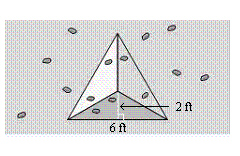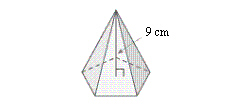Name:    Quiz 6.4-6.6

Multiple Choice
Identify the choice that best completes the statement or answers the question.

Use the net to find the surface area of the regular pyramid.

1.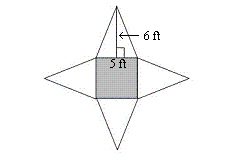a. 60 ftc. 145 ftb. 85 ftd. 120 ft2.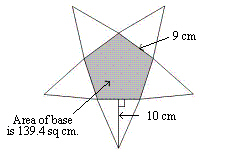a. 225 cmc. 589.4 cmb. 450 cmd. 364.4 cmFind the surface area of the figure.

3.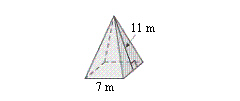a. 357 mc. 203 mb. 308 md. 154 m4.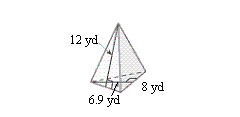a. 171.6 ydc. 315.6 ydb. 288 ydd. 144 yd5.

The surface area of a square pyramid is 95 square feet. The base length is 5 feet. What is the slant height?
 a. 9.5 ft c. 7 ft b. 5 ft d. 3.5 ft

Find the surface area of the figure.

6.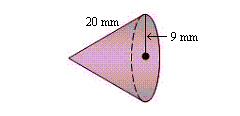a. 565.2 mmc. 813.3 mmb. 1384.7 mmd. 819.5 mm7.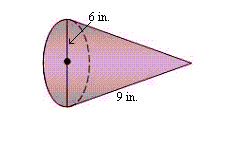a. 113 in.c. 169.6 in.b. 104.5 in.d. 197.8 in.8.

A cone with a diameter of 4 feet and a slant height of 20 inches.
 a. 3315.8 in.c. 4823 in.b. 7360.2 in.d. 3298.3 in.Find the slant heightof the cone.

9.

S = 192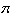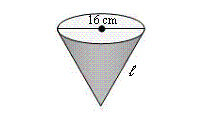a. 16 cm c. 19 cm b. 17 cm d. 2.7 cm

10.

S = 48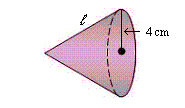a. 5 cm c. 2.4 cm b. 6 cm d. 8 cm

Numeric Response

1.

You are adding a three-sided pyramid to your climbing wall. You make the three lateral faces of the pyramid from a 4-foot by 8-foot sheet of plywood. How many square feet of  plywood are left over?